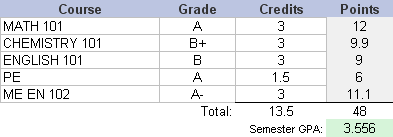Have you ever wondered "How much will my GPA change if I get a B instead of an A," or something like that? With the Vertex42™ GPA Calculator spreadsheet, you can do that type of what-if scenario as well as keep a running record of your grades and cumulative GPA. You can download the GPA Calculator for Excel or OpenOffice, or use Google Sheets. See below for how to calculate your GPA.

categories: Education

for Excel, OpenOffice, and Google Sheets

For: Excel 2007 or later

### Other Versions

License: Private Use (not for distribution or resale)

"No installation, no macros - just a simple spreadsheet" - by

### Description

The Vertex42™ GPA Calculator lets you track your grades from semester to semester, calculating both a semester GPA and your overall cumulative GPA.

You can edit the Grade Scale to define the points associated with letter grades, according to your specific school policy. Then, when you enter the grades into the spreadsheet, the points are automatically totaled and weighted based on the number of credit hours for each course.

New: Version 2.0 of the GPA Calculator lets you evaluate the effect of retaking a course (see the example below). It also includes a separate worksheet for estimating your future GPA.

## Using the GPA Calculator

The GPA Calculator spreadsheet should be pretty intuitive, and some instructions are included at the top of the worksheet.

Adding Semesters: In the Course History worksheet, you can include additional semesters by copying the set of rows for one of the semesters and inserting/pasting the set of rows at the bottom. The formulas in the spreadsheet are designed specifically for making this process simple.

Inserting Rows: If you need to insert rows to add more classes for a particular semester, then make sure to insert new rows above the last row or below the first row in the semester block. This will help ensure that the references in the summation formulas stretch to include the new row(s). You will also need to copy the formula for the Points when you add the new rows.

## How to Calculate GPA

GPA stands for Grade Point Average, but most of the time, to calculate GPA requires using a weighted average. For example, if you earned an A in a 3-credit course and a B in a 2-credit course, your GPA is not simply the average of an A and B. Instead, the grades are weighted based on the number of credit hours in the course. That can seem complicated, but it's not really that bad.

Below are the steps you can use to calculate your GPA if you happen to be caught without a computer.

### Step 1 - Convert Letter Grades to Points

Letter
Points
A+4.3
A4
A-3.7
B+3.3
B3
B-2.7
C+2.3
C2
C-1.7
D+1.3
D1
D-0.7
F0

To the right is a typical 4.0 GPA Scale, showing the grade points and the letter grade equivalent. The first step in calculating your GPA is to convert the letter grades to points.

### Step 2 - Multiply the Points by the Number of Credit Hours

If I got an A- in a 3-credit class, the points for that class would be 3.7 times 3 credits equals 11.1 points. (This is where the weighting comes into play)

### Step 3 - Add the Total Points then Divide by the Total Credit Hours

To calculate your GPA, add up the total grade points and the total credit hours and then divide the total grade points by the total credit hours. If you include only the points and hours for a single semester, this will calculate your GPA for the semester. If you include all the points and hours for every semester, this will calculate your overall cumulative GPA.

The figure below shows an example of a GPA calculation for a semester in college.## Retaking a Course

Many programs only allow a certain number of failing or D grades, or you may need to have a certain GPA to qualify. Sometimes you have no choice but to retake a class, but it can also be one of the fastest ways to increase your overall GPA. The new version of the GPA calculator lets you estimate what effect retaking a class can have on your GPA. It assumes that a retaken course replaces your previous grade.

For example, let's say that you've attempted 50 credit hours and earned a total of 150 points, so your GPA is currently a 3.00. Maybe for some reason you'd failed one of your 3-credit classes. If you were to retake that class and get an A, your new GPA might be a 3.24 (depending on the grading scale). That is a huge jump for just one class! If you didn't retake that class, it would take 5 more A's in 3-credit classes to reach a GPA of 3.23.

### Custom GPA Calculators

• GPA Calculator for RCC - A custom Google Sheets version of the GPA Calculator created for Robeson Community College's allied health program.

### References and Resources

• Grade Point Average (GPA) at wikipedia.com - General information and GPA scales used around the world.